# The work of Batch Norm was overthrown by MIT researchers in 2018

Share
/ 2019-07-19 / News

Batch Normalization was introduced by Google in 2015 and is widely used because it speeds up training and reduces the sensitivity of learning rates.

But the interpretation of Batch Norm's working principle in the paper was overturned by researchers inMIT in 2018, although this paper It has been proposed in 2018, but I believe that there are still many people like me. When I read related blogs and papers online, most of the content was written before the paper was presented.

Now DL has gradually become experimental science. Generally, after discovering the improvement of performance, it analyzes the cause of it, but the idea of this paper is very good. From a series of data, formulas and other perspectives, it is analyzed why batch Normalization works.

batch Normalization's proposal

Abandoning Norm, we often normalize the input when doing feature engineering, otherwise if a certain feature number is special Large (for example, a feature is 0-1, a feature is 0-1000), and the second feature is likely to have a large impact on the overall model parameters. Or, when the distribution of training set data is inconsistent, For example, the range of features of the previous input is 0-1, and the latter is 0-100. The training effect of the network is not good.

So, As many people have said, we hope that in the deep learn, the data flowing through the entire network is independently and identically distributed.

Why do you need to be independent and identically distributed?

I think this can be explained in two ways.

On the one hand, we hope to train the training set and get good performance on the test set. Then we need to ensure that the training set and the test set are from the same space, exactly the same distribution, which puts forward the same distribution. In addition, although the data comes from the same space, we don't want all the data to gather in a small space in the space, but hope that all the data is representative of the whole space, so in the process of sampling We want to be able to sample all the data independently.

On the other hand, it is actually another point to explain the last paragraph, if the data is not the same distribution, for example, all the features of sample 1 Between 0-1 and sample 2 at 10-100, the parameters of the network are actually difficult to cater to both types of data.

What did batch Normalization do?

The above mentioned that when the data came in, it was hoped that it would be independent and identically distributed. But the author of batch normalization doesn't think it's enough. It should be processed once in each layer of deep learning to ensure that it is equally distributed on each layer.

He thinks this way: Suppose the network has n layers, the network is training, and there is no convergence. At this time, x1 is input, after the first layer, but the first layer has not learned the correct weight, so after the matrix multiplication of weight, the number of the second layer will not be messy? Will some node values in the second layer be single digits, and some node values are hundreds of? If you think about it, it is quite possible. The internal parameters are randomly initialized, so the result is really hard to say. Then the horrible thing came, and the second layer of these chaotic numbers went to the third floor. The input of the third layer was the number of chaos, the output is of course not good, and so on.

So there are two main problems:

1. So there is no network in front When converging, the latter network actually can't learn anything. The bottom of a building is swaying, and the top is not good. Therefore, it is necessary to wait for the previous layer to converge, and the training of the latter layer has an effect.

2. Because in general, each layer inside the network needs to add a layer of activation to increase the nonlinearity, then if the value is larger, it will be activated after activation. On the S curve, it will be closer to 0 or 1, the gradient is small, and the convergence will be slow.

So batch Normalization wants to add a norm to each layer to standardize, so that the number distribution of each layer is the same, and becomes the mean value of 0, the standard distribution of variance 1 . The normalized formula of the Gaussian distribution is the part of the brackets in the following formula. The value is subtracted from the mean and divided by the variance to give a mean of 0.A standard normal distribution with a variance of 1. As for γ and β, it is two parameters that need to be learned. γ further scales the variance of the data, and β produces an offset to the mean of the data.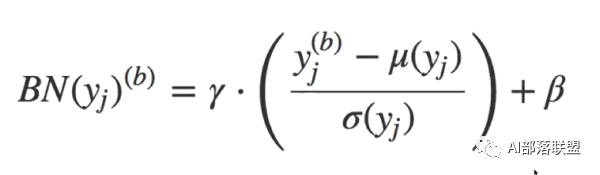Why do you normalize to mean 0, and after variance 1, do you want to modify the variance and mean? Does normalization still make sense?

This is because we can't guarantee what the characteristics of this layer of network are learned. If it is simply normalized, it is likely to be destroyed. For example, the S-type activation function, if the feature learned at this level is at the top of S, then after normalization, we force the feature to the middle of S, and the feature is destroyed. Note that γ and β are trained parameters and each layer is different.So for the actual situation of each layer, it will try to restore the features learned by this layer of network.

Results

Using the VGG network, CIFAR10 dataset (below), you can see Compared with no:

1. The accuracy of the pre-training period is high, that is, the convergence is faster.

2. Reduce the sensitivity to learning rate. Figure 2 shows the direct oscillation of the network without norm when lr=0.5 However, the addition of nrom still performed well.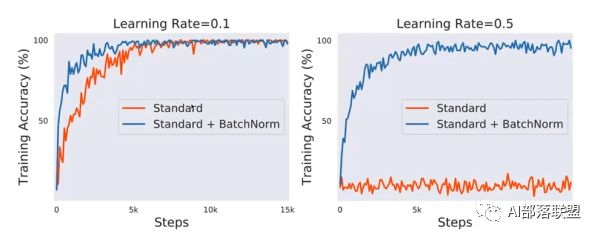So the principle of batch normalization is not very deep, but a standardization is added to each layer to make the data share the same, and then its variance and mean A transformation is performed to recover the features captured by the layer. Finally, two major changes are made, first the convergence is faster, and secondly the sensitivity to lr is reduced.

that Every layer in the network is normalized. Is it a simple idea that has not been used? Is it a researcher?

I believe there are many Researcher has tried to re-standardize all layers, but I believe that the final effect is not very good, because many features are re-modified to mean 0 variance 1, the feature information will be lost. So the author's theoretical breakthrough is in one. After using γ and β, the data distribution was re-modified to recover the missing features. Of course,Just let these two parameters be self-learning.

In fact, most of the explanations that bloggers use today are all of the above. Including I have always thought this is the case.

But the "How Does Batch Normalizetion Help Optimization" paper argues that the network convergence after using norm is faster, lr The lower sensitivity is correct, but not because of the reason stated in the paper, but because the standardization of each layer makes the final loss function become a smooth surface and the final performance is improved. Let's explain the idea:

Batch Normalization explains the rebuttal

Experimental test

MIT researchers did not present their own explanations at the beginning of the paper. Instead, if the original author is talking about Then, we will first verify according to the original author's idea (because two papers are involved, this article will write the author of batch normalization as the original author, and the author of How Does Batch Normalizetion Help Optimization is written as a researcher from MIT) :

The original author believes that because the layers in the network are standardized, the distribution is the same, so the performance is improved.

Researchers from MIT did three experiments for comparison: ordinary networks that don't use norm, regular networks that use nrom, and Norm networks that add noise.

Norm network adds noise to the performance that the original author believes is the same distribution. Then the researchers from MIT on the Norm network, manually add noise to each layer, so that the third network Norm is used, but the distribution of each layer has been disrupted and no longer satisfies the same distribution.

The experimental results in the following figure show that even the nrom network Noise has been added, but performance is still similar to that of adding a norm network.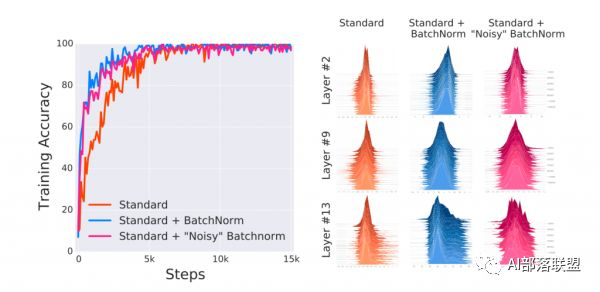The reason for the final performance improvement is definitely not the interpretation of the same data distribution, which means that the explanation given by the original author is wrong. There must be other reasons.

Intuitive understanding of the new interpretation of batch Normalization

In basic learning, we all know The loss function is expected to be smooth when designing the loss, so that we can smoothly use the gradient drop to update the parameters and find a better advantage. But many times loss is not as good as we think. For example, in the figure below, the left and right sides are loss functions, but the loss on the left is continuous, but not smooth. It is difficult to ensure the effectiveness and rapidity of the decline during the gradient descent process, and it is also easy to fall into the local optimal solution. While the graph on the right has a local optimal solution at the four vertices, it is still a very good loss overall.

MIT researchers believe that the effectiveness of batch normalization is that it turns the original loss on the left into the loss on the right.Caused the two results mentioned above:

1. Convergence speed changes.

2. The learning rate setting is no longer so sensitive.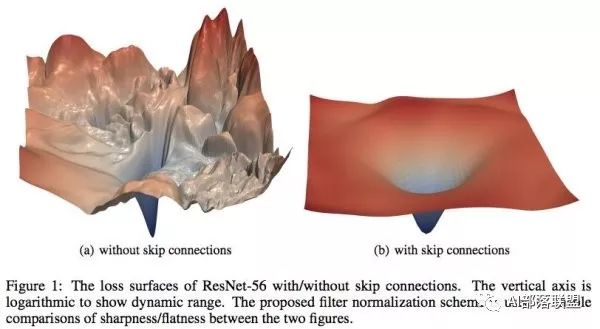Analyze

MIT researchers define two functions:

The first is the calculation of the value of loss, which is the first formula below, the internal loss is the current loss, then the loss is derived, multiplied by the learning rate, x is the internal parameter,Make an update to the parameters, then put the new parameters into the loss function and calculate the current loss value. Don't look at this formula to look a bit around, in fact, is to find the current loss value.

The second formula is to calculate the gradient difference of loss, or as a secondary derivation of loss (I feel).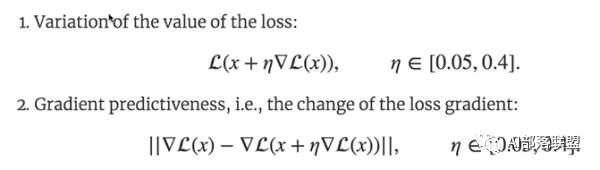The reason why these two formulas are proposed in the paper is to get two quantities.

The first formula can get the loss in the training process, then take the loss of each step and draw it into a curve, which can be considered as loss Volatility.I think that if a loss function itself is relatively smooth, then the loss value obtained by the first formula does not have a large floating.

The second amount is the gradient difference for calculating the loss. It can be considered whether the direction change will be larger when the hill is going in the downward direction. In other words, a better loss function, in each step, the gradient obtained by loss should not be too large. It’s like going down from the mountain, we generally don’t change too much in the direction of the moment, instead of walking down smoothly (the gradient of the loss is stable), suddenly there is a cliff in front (the gradient of the loss changes suddenly) Instead, there should be a step (the gradient of the gradient changes smoothly).

MIT researchers will use norm and a common network to compare these two volumes:You can see the network that does not use norm (red), the gradient difference of the loss is Larger. The network using norm (blue) floats very little. It also proves from the side that the loss of the ordinary network tends to the left, while the use of norm, the loss tends to the right.The above two formulas are actually the core of the whole paper. MIT's researcher smoke is based on these two quantities and thus refuted the original text.

1. The L-Lipschitz constant is used in the paper to quantify the smoothness of loss (that is, the dry matter of Equation 1), which limits the smoothness of loss. (The following definition is from Baidu Encyclopedia)

L-Lipschitz: Intuitively, the Lipschitz continuous function limits the speed of function changes, in line with Lipschitz The slope of the conditional function must be less than a real number called the Lipschitz constant (this constant depends on the function). For functions in a subset of real sets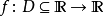,If there is a constant K, make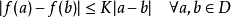, then f is in accordance with the Lipschitz condition, and the minimum constant k for f is called Lipsch Constant.

I personally think that it is a change in the way that the first derivative of the limit loss is less than the constant k. The first derivative shows on the curve that the slope must be less than one value, that is, the slope cannot be too large. Under the constraints of this condition, even if the surface of the loss falls, it is very slow.

2. The paper uses another stronger smooth condition to limit:--smoothness (that is, the work of Formula 2).

β-smoothness limits the slope of the loss slope (which can be thought of as a slope difference) not to exceed a certain value.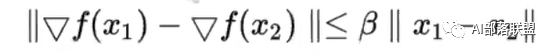I think this is essentially a second-order derivation. A limit is imposed on the result of the second-order derivation, so that the function is still a smoother function that does not greatly mutate under the second-order condition.

It is also because of the use of batch norm network,Its loss changed from the original original rugged state to a condition that satisfies the two strong conditions of L-Lipschitz and β-smoothness, enabling the bath norm to work.

summary

The original author proposed batch norm and caused two results:

1. Convergence is faster.

2. It is less sensitive to the learning rate (that is, whether the lr setting is reasonable does not affect the final result to a large extent).

and believe that each layer in the network has been distributed once, which is the main cause of the results.

MIT researchers based on this idea, will use Norm's network to randomly add Gaussian noise, so that the network adds norm and removes the same distribution effect brought by norm, but the results show that the advantage still exists, so the original author The explanation was refuted.

The MIT researchers then used the first-order information and second-order information of the loss to evaluate and found that the loss after using norm was Both the first order and the second order have good properties, so it is inferred that the effect of nrom can not be explained by the original author, but because norm directly acts on the loss function, and the loss function becomes a first order. Second-order smoothing function.

Keywords: MIT paper
Relevant articles recommend

Top suppliers

Product:Photo Coupler,Optocoupler,IC,TOS,ON,AVAGO,SHARP,RENESAS,CT,EL,LTV,TI,NXP,ST
1YRS
Product:Infrared Receiver Module,Infrared Emitting Diode,Silicon PIN Photodiode,Silicon Phototransistor,LED,Silicon Photocell,Hall Effect IC,Photoelectric switch,Photoelectric encoder,IC.
1YRS
Product:KSD301 KSD9700 thermostat thermal protector fuse sensor
1YRS
Product:IC,PCB,module,capacitor,resistor,diodes,LED,LCD
1YRS
Product:Electronics components，ICs，MCU，RS-485/RS-422 Transceiver
9YRS
Subscribe News

Enter your email address:

Hot News

Contact Us

+86-755-83536845

One to One Customer Service

17190417227